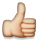Welcome to the Linux Foundation Forum!

# Function context scope doubt

Hi everybody,
Hope you all are doing great.

I have a question regarding how object's context works on functions at Node.JS.
For example:

```function nestedFunctions() {
var a = "I am a variable"
console.log("Initial context:")
console.log(this)

function one() {
console.log("First nested function, this is my this context:")
console.log(this)
console.log("First nested function, this is my a value:")
console.log(a)

function two() {
console.log("Second nested function, this is my this context:")
console.log(this)
console.log("Second nested function, this is my a value:")
console.log(a)
}
two()
}
one()
}
nestedFunctions()
```

The output of that code is:

```Initial context:
Object Global
First nested function, this is my this context:
Object Global
First nested function, this is my a value:
I am a variable
Second nested function, this is my this context:
Object Global
Second nested function, this is my a value:
I am a variable
```

Everything OK so far, because the function context (this) is created on global scope, and so the nested functions. But my doubt is when running the following code:

```class Dog {
constructor(name) {
this.name = name
}

bark() {
console.log(`Woof, my name is \${this.name}`);
}
nestedFunctions() {
var a = "I am a variable"
console.log("Initial context:")
console.log(this)

function one() {
console.log("First nested function, this is my this context:")
console.log(this)
console.log("First nested function, this is my a value:")
console.log(a)

function two() {
console.log("Second nested function, this is my this context:")
console.log(this)
console.log("Second nested function, this is my a value:")
console.log(a)
}
two()
}
one()
}
}

let rex = new Dog('Rex')
//rex.sitAndBark()
rex.nestedFunctions()
```

Output:

```Initial context:
Dog { name: 'Rex' }
First nested function, this is my this context:
undefined
First nested function, this is my a value:
I am a variable
Second nested function, this is my this context:
undefined
Second nested function, this is my a value:
I am a variable
```

I am having troubles understanding that last one, and why the this of the nested functions is undefined. Why isn't the global context instead of undefined (like when declared outside a class, i.e, the first example)?

Best regards to all

• Another example with the function defined on an object:

```var rex = {
nestedFunctions() {
var a = "I am a variable"
console.log("Initial context:")
console.log(this)

function one() {
console.log("First nested function, this is my this context:")
console.log(this)
console.log("First nested function, this is my a value:")
console.log(a)

function two() {
console.log("Second nested function, this is my this context:")
console.log(this)
console.log("Second nested function, this is my a value:")
console.log(a)
}
two()
}
one()
}
}

rex.nestedFunctions()
```

The output in that case is:

```Initial context:
{ nestedFunctions: [Function: nestedFunctions] }
First nested function, this is my this context:
Object Global
First nested function, this is my a value:
I am a variable
Second nested function, this is my this context:
Object Global
Second nested function, this is my a value:
I am a variable
```

Which is the same behaviour of the first example. I don't get why in the second case, the one on a class definition, the result of the nested "this" is undefined instead of the global context.

• @IgnacioAcuna Exactly correct